# Tutorial: Linear Regression – Time variables and shifts (#2)

#### Part 2. Simple multifactorial linear regression

In the previous part of this tutorial, we cleaned the data file from the measuring station. A new, completed measurement data file has been created, which we will now open.

We will now continue to prepare data for further analysis. One of the most important variables describing in linear regression is time. Most artificial and natural phenomena operate in hourly, daily and monthly cycles.

In :
```import pandas as pd
```
Out:
Unnamed: 0 Date Time CO(GT) PT08.S1(CO) C6H6(GT) PT08.S2(NMHC) NOx(GT) PT08.S3(NOx) NO2(GT) PT08.S4(NO2) PT08.S5(O3) T RH AH
0 0 10/03/2004 18.00.00 2.6 1360.0 11.9 1046.0 166.0 1056.0 113.0 1692.0 1268.0 13.6 48.9 0.7578
1 1 10/03/2004 19.00.00 2.0 1292.0 9.4 955.0 103.0 1174.0 92.0 1559.0 972.0 13.3 47.7 0.7255
2 2 10/03/2004 20.00.00 2.2 1402.0 9.0 939.0 131.0 1140.0 114.0 1555.0 1074.0 11.9 54.0 0.7502

## Step 1. Launching the time variable

We check what the date format is

In :
```df[['Date','Time']].dtypes
```
Out:
```Date    object
Time    object
dtype: object```

There is no date format in dataframe. Link columns containing time.

In :
```df['DATE'] = df['Date']+' '+df['Time']
```
Out:
```0    10/03/2004 18.00.00
1    10/03/2004 19.00.00
2    10/03/2004 20.00.00
3    10/03/2004 21.00.00
4    10/03/2004 22.00.00
Name: DATE, dtype: object```

We create a new column containing the date and time. Then we convert the object format to the date format.

In :
```df['DATE'] = pd.to_datetime(df.DATE, format='%d/%m/%Y %H.%M.%S')
df.dtypes
```
Out:
```Unnamed: 0                int64
Date                     object
Time                     object
CO(GT)                  float64
PT08.S1(CO)             float64
C6H6(GT)                float64
PT08.S2(NMHC)           float64
NOx(GT)                 float64
PT08.S3(NOx)            float64
NO2(GT)                 float64
PT08.S4(NO2)            float64
PT08.S5(O3)             float64
T                       float64
RH                      float64
AH                      float64
DATE             datetime64[ns]
dtype: object```

# Step 2. We add more columns based on the time variable

In industry, the day of the week is very important, so in such models it is worth adding a column with the number of the day.

In :
```df['Month'] = df['DATE'].dt.month
df['Weekday'] = df['DATE'].dt.weekday
df['Weekday_name'] = df['DATE'].dt.weekday_name
df['Hours'] = df['DATE'].dt.hour
```
In :
```df[['DATE','Month','Weekday','Weekday_name','Hours']].sample(3)
```
Out:
DATE Month Weekday Weekday_name Hours
6109 2004-11-20 07:00:00 11 5 Saturday 7
3537 2004-08-05 03:00:00 8 3 Thursday 3
8053 2005-02-09 07:00:00 2 2 Wednesday 7

#### Graphical analysis of pollution according to time variables

In :
```df.pivot_table(index='Weekday_name', values='CO(GT)', aggfunc='mean').plot(kind='bar')
```
Out:
`<matplotlib.axes._subplots.AxesSubplot at 0x12f20bbdb38>`
In :
```df.pivot_table(index='Month', values='CO(GT)', aggfunc='mean').plot(kind='bar')
```
Out:
`<matplotlib.axes._subplots.AxesSubplot at 0x12f20f02320>`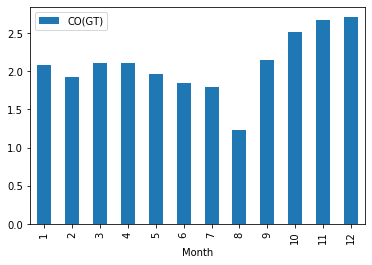In :
```df.pivot_table(index='Hours', values='CO(GT)', aggfunc='mean').plot(kind='bar')
```
Out:
`<matplotlib.axes._subplots.AxesSubplot at 0x12f210e77f0>`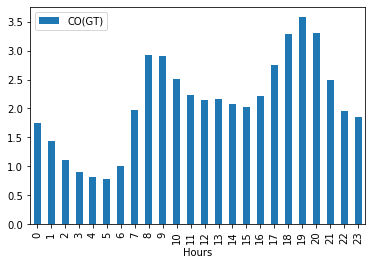# Step 3. Correlation analysis¶

we set the result variable as:

#### CO(GT) – actual hourly average CO concentration in mg / m^3 (reference analyzer)

In :
```del df['Unnamed: 0']
```
In :
```CORREL = df.corr()
PKP = CORREL['CO(GT)'].to_frame().sort_values('CO(GT)')
PKP
```
Out:
CO(GT)
PT08.S3(NOx) -0.715683
Weekday -0.140231
RH 0.020122
AH 0.025227
T 0.025639
Month 0.112291
Hours 0.344071
PT08.S4(NO2) 0.631854
NO2(GT) 0.682774
NOx(GT) 0.773677
PT08.S5(O3) 0.858762
PT08.S1(CO) 0.886114
PT08.S2(NMHC) 0.918386
C6H6(GT) 0.932584
CO(GT) 1.000000
In :
```import matplotlib.pyplot as plt

plt.figure(figsize=(10,8))
PKP.plot(kind='barh', color='red')
plt.title('Correlation with the resulting variable: CO ', fontsize=20)
plt.xlabel('Correlation level')
plt.ylabel('Continuous independent variables')
```
Out:
`Text(0, 0.5, 'Continuous independent variables')`
`<Figure size 720x576 with 0 Axes>`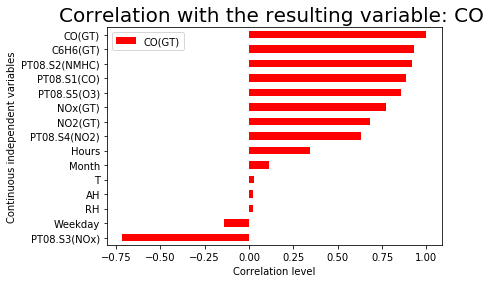Variables based on time are not very well correlated with the described variable: CO (GT).
The temptation arises to use better, better correlated independent variables for the model. The problem is that these variables can be part of the result. So if there is pollution then all substances are in the air.

Our task is to examine how weather and time affect the level of pollution. We’ll cover this task in the next part of the tutorial.

## Step 4. We are now checking shift

for independent variables with low direct correlation.
How does weather affect CO2 levels?

### Variable RH – Relative humidity (%)

We check a variable with very low correlation with the resulting CO (GT) variable

In :
```def cross_corr(x, y, lag=0):
return x.corr(y.shift(lag))

def shift_Factor(x,y,R):
x_corr = [cross_corr(x, y, lag=i) for i in range(R)]

# R factor is the number of the shifts who should be checked by the function
Kot = pd.DataFrame(list(x_corr)).reset_index()
Kot.rename(columns={0:'Corr', 'index':'Shift_num'}, inplace=True)

# We find optimal correlation shift
Kot['abs'] = Kot['Corr'].abs()
SF = Kot.loc[Kot['abs']==Kot['abs'].max(), 'Shift_num']
p1 = SF.to_frame()
SF = p1.Shift_num.max()

return SF
```
In :
```x = df.RH       # independent variable
y = df['CO(GT)']    # dependent variable
R = 20           # number of shifts who will be checked
```
In :
```SKO = shift_Factor(x,y,R)
print('Optimal shift for RH: ',SKO)
```
```Optimal shift for RH:  12
```
In :
```cross_corr(x, y, lag=SKO)
```
Out:
`0.39204313671898056`

### Variable AH – Absolute humidity

We check a variable with very low correlation with the resulting CO (GT) variable

In :
```x = df.AH       # independent variable
SKP = shift_Factor(x,y,R)
print('Optimal shift for AH: ',SKP)
```
```Optimal shift for AH:  12
```
In :
```cross_corr(x, y, lag=SKP)
```
Out:
`0.043756364102677595`

Absolute humidity AH does not correlate with the variable CO (GT) so we eliminate it from the model

### Variable: Temperature in ° C¶

We check a variable with very low correlation with the resulting CO (GT) variable.

In :
```x = df['T']      # independent variable
PKP = shift_Factor(x,y,R)
print('Optimal shift for T: ',PKP)
```
```Optimal shift for T:  12
```
In :
```cross_corr(x, y, lag=PKP)
```
Out:
`-0.22446569561762522`

### We are now creating a new DataFrame with a 12-hour shift

It turns out that temperature and humidity only correlate after 12 hours from the time the CO contamination changes.
Data shift creation function.

In :
```def df_shif(df, target=None, lag=0):
if not lag and not target:
return df
new = {}
for h in df.columns:
if h == target:
new[h] = df[target]
else:
new[h] = df[h].shift(periods=lag)
return  pd.DataFrame(data=new)
```

Our goal is to create a multiple regression model:

``````- Independent variables are: Temperature (T) and Relative humidity RH (%)
- The dependent variable is the level of CO (GT)``````
In :
```df2 = df[['DATE', 'CO(GT)','RH', 'T']]
```

Adds a date and time to record temperature and humidity.

In :
```df2['weather_time'] = df2['DATE']
```
```C:ProgramDataAnaconda3envsOLD_TFlibsite-packagesipykernel_launcher.py:1: SettingWithCopyWarning:
A value is trying to be set on a copy of a slice from a DataFrame.
Try using .loc[row_indexer,col_indexer] = value instead

See the caveats in the documentation: http://pandas.pydata.org/pandas-docs/stable/user_guide/indexing.html#returning-a-view-versus-a-copy
"""Entry point for launching an IPython kernel.
```
In :
```df2.head(3)
```
Out:
DATE CO(GT) RH T weather_time
0 2004-03-10 18:00:00 2.6 48.9 13.6 2004-03-10 18:00:00
1 2004-03-10 19:00:00 2.0 47.7 13.3 2004-03-10 19:00:00
2 2004-03-10 20:00:00 2.2 54.0 11.9 2004-03-10 20:00:00
In :
```df3 = df_shif(df2, 'weather_time', lag=12)
df3.rename(columns={'weather_time':'Shift_weather_time'}, inplace=True)
```
Out:
DATE CO(GT) RH T Shift_weather_time
0 NaT NaN NaN NaN 2004-03-10 18:00:00
1 NaT NaN NaN NaN 2004-03-10 19:00:00
2 NaT NaN NaN NaN 2004-03-10 20:00:00
3 NaT NaN NaN NaN 2004-03-10 21:00:00
4 NaT NaN NaN NaN 2004-03-10 22:00:00
5 NaT NaN NaN NaN 2004-03-10 23:00:00
6 NaT NaN NaN NaN 2004-03-11 00:00:00
7 NaT NaN NaN NaN 2004-03-11 01:00:00
8 NaT NaN NaN NaN 2004-03-11 02:00:00
9 NaT NaN NaN NaN 2004-03-11 03:00:00
10 NaT NaN NaN NaN 2004-03-11 04:00:00
11 NaT NaN NaN NaN 2004-03-11 05:00:00
12 2004-03-10 18:00:00 2.6 48.9 13.6 2004-03-11 06:00:00
In :
```df4 = df_shif(df3, 'RH', lag=12)
df4.rename(columns={'RH':'Shift_RH'}, inplace=True)
```
In :
```df5 = df_shif(df4, 'T', lag=12)
df5.rename(columns={'T':'Shift_T'}, inplace=True)
```

Deletes rows with incomplete data.

In :
```df5 = df5.dropna(how ='any')
```
In :
```df5.head()
```
Out:
DATE CO(GT) Shift_RH Shift_T Shift_weather_time
36 2004-03-10 18:00:00 2.6 58.1 10.5 2004-03-11 06:00:00
37 2004-03-10 19:00:00 2.0 59.6 10.2 2004-03-11 07:00:00
38 2004-03-10 20:00:00 2.2 57.4 10.8 2004-03-11 08:00:00
39 2004-03-10 21:00:00 2.2 60.6 10.5 2004-03-11 09:00:00
40 2004-03-10 22:00:00 1.6 58.4 10.8 2004-03-11 10:00:00

The table can be understood as meaning that a specific temperature at 6:00 gives a specific concentration of carbon monoxide at 18:00.

### Graphical analysis of relationships – Humidity and temperature to carbon monoxide

It looks rather poor

In :
```import matplotlib.pyplot as plt

df5.plot(x='Shift_T', y='CO(GT)', style='o')
plt.title('Shift_T vs CO(GT)')
plt.xlabel('Shift_T')
plt.ylabel('CO(GT)')
plt.show()
```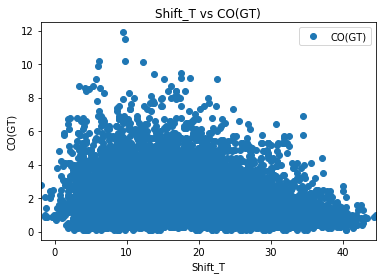In :
```df5.plot(x='Shift_RH', y='CO(GT)', style='o')
plt.title('Shift_RH vs CO(GT)')
plt.xlabel('Shift_RH')
plt.ylabel('CO(GT)')
plt.show()
```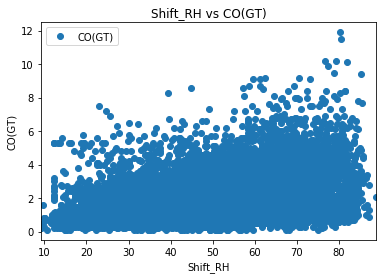## Step 5. Building a multiple linear regression model in Sklearn

Declares X, y variables into the model.

In :
```X = df5[['Shift_RH', 'Shift_T']].values
y = df5['CO(GT)'].values
```

I divide the collection into training variables and test variables.

In :
```from sklearn.model_selection import train_test_split
from sklearn.linear_model import LinearRegression

X_train, X_test, y_train, y_test = train_test_split(X, y, test_size=0.2, random_state=0)
```

I am building a regression model.

In :
```regressor = LinearRegression()
regressor.fit(X_train, y_train)
```
Out:
`LinearRegression(copy_X=True, fit_intercept=True, n_jobs=None, normalize=False)`
In :
```import numpy as np

y_pred = regressor.predict(X_test)
y_pred = np.round(y_pred, decimals=2)
```

Comparison of variables from the model with real variables.

In :
```dfKK = pd.DataFrame({'CO(GT) Actual': y_test, 'CO(GT)_Predicted': y_pred})
```
Out:
CO(GT) Actual CO(GT)_Predicted
0 0.5 1.63
1 1.9 1.91
2 3.4 2.40
3 1.2 1.45
4 2.4 2.40
In :
```from sklearn import metrics

```
Out:
`<matplotlib.axes._subplots.AxesSubplot at 0x12f2b9a5898>`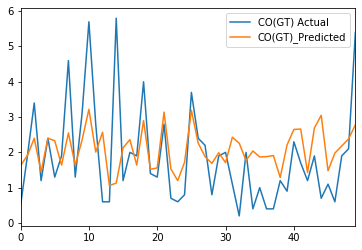In :
```from sklearn import metrics

print('Mean Absolute Error:    ', metrics.mean_absolute_error(y_test, y_pred))
print('Mean Squared Error:     ', metrics.mean_squared_error(y_test, y_pred))
print('Root Mean Squared Error:', np.sqrt(metrics.mean_squared_error(y_test, y_pred)))
```
```Mean Absolute Error:     1.0011099195710456
Mean Squared Error:      1.779567238605898
Root Mean Squared Error: 1.3340042123643756
```
In :
```print('Mean Squared Error:     ', metrics.r2_score(y_test, y_pred))
```
```Mean Squared Error:      0.15437562015505324
```

Carbon monoxide contamination cannot be predicted based on humidity and temperature.
In the next part, we will continue the analysis and preparation of data for linear regression.